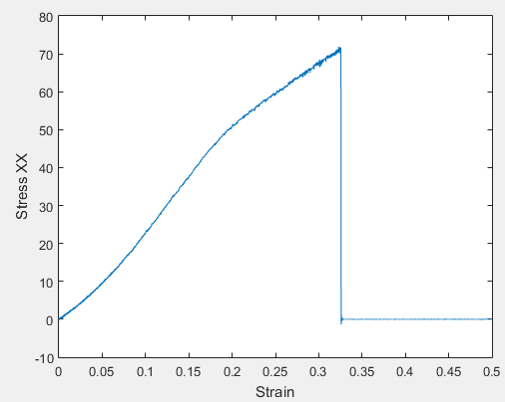# Correct elastic, lattice, and P.E. but incorrect stress strain curve

Dear Ray, and LAMMPS users,

Hi,

I’m using LAMMPS to simulate the failure behavior of my ceramic system. To make sure of the validity of structure, potential and etc I first tried to calculate the elastic constants using the codes that is available in Example directory. By doing so, I obtained the correct value for elastic constants. I also checked the lattice constants, and even P.E. of the structure and compared it with the literature and all of the works fine. Up to here everything was calculated in ( P P P ) as bulk material. Then I started a new simulation using ( P P S ) because I want to work on nanomembrane ( Finite thickness in z ). Then I used the below code:

#INITIALIZATION
units metal
boundary p p s
atom_style atomic
#atom_modify map array
#box tilt large
pair_style tersoff
pair_coeff * * SiN.Tersoff N Si

# ------------------------- Minimization ---------------------------------

#replicate 10 6 5
compute stress all stress/atom NULL
compute stress1 all reduce sum c_stress
compute stress2 all reduce sum c_stress
compute stress3 all reduce sum c_stress
compute stress4 all reduce sum c_stress
compute stress5 all reduce sum c_stress
compute stress6 all reduce sum c_stress

thermo 1
thermo_style custom step lx ly lz press pxx pyy pzz pxy pxz pyz pe temp
fix 2 all box/relax x 0 y 0 vmax 0.001
min_style sd
minimize 1.0e-25 1.0e-25 100000 100000

unfix 2
write_data Min.in

# ------------------------- Equilibration ( NPT ) ---------------------------------

reset_timestep 0
velocity all create 100 277387
fix 1 all npt temp 100 300 0.1 x 0 0 1.0 y 0 0 1.0 drag 0.3
thermo 1000
thermo_style custom step lx ly lz press pxx pyy pzz pxy pxz pyz pe temp
dump q2 all custom 1000 dump.npt.*.cfg id type xs ys zs c_stress c_stress c_stress c_stress c_stress c_stress
#dump_modify q2 element N Si
run 100000
undump q2
unfix 1
write_data EQ2.in

# ------------------------- Deformation ---------------------------------

reset_timestep 0
variable tmp equal “lx”
variable L0 equal {tmp} print "Initial Length, L0: {L0}"
fix 1 all npt temp 300 300 0.1 y 0 0 1.0 drag 0.3
fix 2 all deform 1 x erate 0.0001 units box remap x
variable strain equal “(lx - v_L0)/v_L0”
variable p1 equal “v_strain”
variable p2 equal “-pxx/10000”
variable p3 equal “-pyy/10000”
variable p4 equal “-pzz/10000”
variable p5 equal “-pxy/10000”
variable p6 equal “-pxz/10000”
variable p7 equal “-pyz/10000”
thermo 5000
thermo_style custom step v_strain temp v_p1 v_p2 v_p3 v_p4 v_p5 v_p6 v_p7 ke pe press
dump 1 all custom 5000 dump.tensile.*.cfg id type xs ys zs c_stress c_stress c_stress c_stress c_stress c_stress
#dump_modify 1 element N Si
fix def1 all print 500 “{p1} {p2} {p3} {p4} {p5} {p6} \${p7}” file Final.dat screen no
run 150000000
write_data Def.in

But, the stress strain curve that I obtain is not in good agreement with Literature, especially the stress value at fracture point is too high. As you can see I used -Pxx as the true stress. I wonder if you could please help me out with it.

Thanks,

Ali